# Print words of a string in reverse order

• Difficulty Level : Medium
• Last Updated : 21 Jun, 2022

Let there be a string say “I AM A GEEK”. So, the output should be “GEEK A AM I” . This can done in many ways. One of the solutions is given in Reverse words in a string .

Examples:

```Input : I AM A GEEK
Output : GEEK A AM I

Input : GfG IS THE BEST
Output : BEST THE IS GfG```

This can be done in more simpler way by using the property of the “%s format specifier” .
Property: %s will get all the values until it gets NULL i.e. ‘\0’.

Example: char String[] = “I AM A GEEK” is stored as shown in the image below :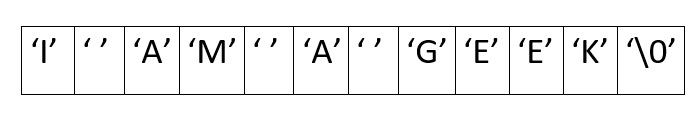Approach: Traverse the string from the last character, and move towards the first character. While traversing, if a space character is encountered, put a NULL in that position and print the remaining string just after the NULL character. Repeat this until the loop is over and when the loop ends, print the string, the %s will make the printing of characters until it encounters the first NULL character.

Let us see the approach with the help of diagrams:
step 1: Traverse from the last character until it encounters a space character .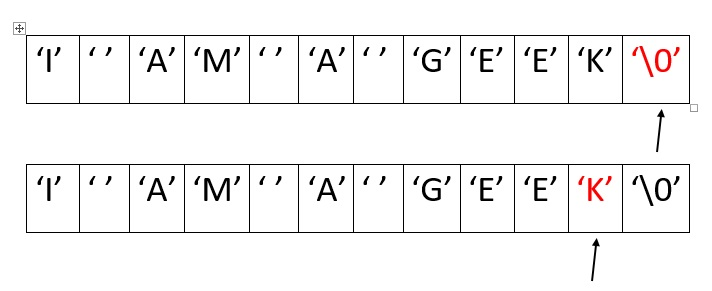Step 2: Put a NULL character at the position of space character and print the string after it.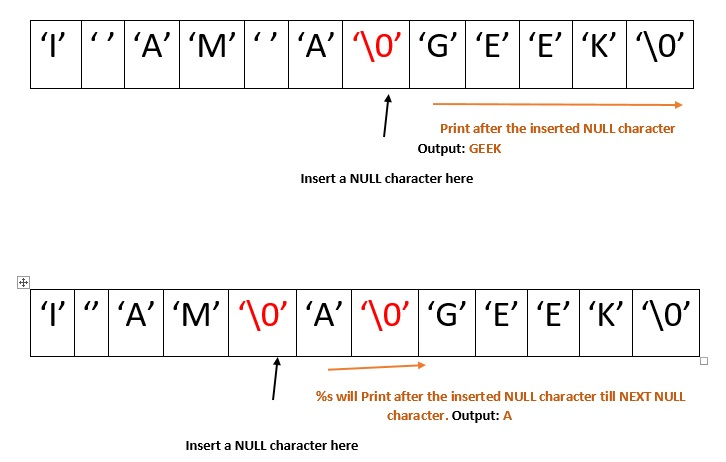Step 3: At the end, the loop ends when it reaches the first character, so print the remaining characters, it will be printed the first NULL character, hence the first word will be printed.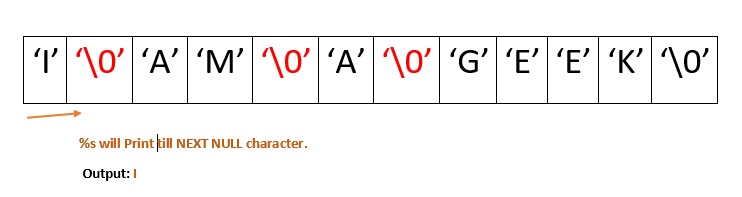## C++

 `// C++ program to print reverse``// of words in a string.``#include ` `using` `namespace` `std;` `string wordReverse(string str)``{``    ``int` `i = str.length() - 1;``    ``int` `start, end = i + 1;``    ``string result = ``""``;` `    ``while` `(i >= 0) {``        ``if` `(str[i] == ``' '``) {``            ``start = i + 1;``            ``while` `(start != end)``                ``result += str[start++];` `            ``result += ``' '``;` `            ``end = i;``        ``}``        ``i--;``    ``}``    ``start = 0;``    ``while` `(start != end)``        ``result += str[start++];` `    ``return` `result;``}` `// Driver code``int` `main()``{``    ``string str = ``"I AM A GEEK"``;` `    ``cout << wordReverse(str);` `    ``return` `0;``}` `// This code is contributed``// by Imam`

## C

 `// C program to print reverse of words in``// a string.``#include ``#include ` `void` `printReverse(``char` `str[])``{``    ``int` `length = ``strlen``(str);` `    ``// Traverse string from end``    ``int` `i;``    ``for` `(i = length - 1; i >= 0; i--) {``        ``if` `(str[i] == ``' '``) {` `            ``// putting the NULL character at the``            ``// position of space characters for``            ``// next iteration.``            ``str[i] = ``'\0'``;` `            ``// Start from next character``            ``printf``(``"%s "``, &(str[i]) + 1);``        ``}``    ``}` `    ``// printing the last word``    ``printf``(``"%s"``, str);``}` `// Driver code``int` `main()``{``    ``char` `str[] = ``"I AM A GEEK"``;``    ``printReverse(str);``    ``return` `0;``}`

## Java

 `// Java program to print reverse``// of words in a string.``import` `java.io.*;``import` `java.lang.*;``import` `java.util.*;` `class` `GFG {``    ``static` `String wordReverse(String str)``    ``{``        ``int` `i = str.length() - ``1``;``        ``int` `start, end = i + ``1``;``        ``String result = ``""``;` `        ``while` `(i >= ``0``) {``            ``if` `(str.charAt(i) == ``' '``) {``                ``start = i + ``1``;``                ``while` `(start != end)``                    ``result += str.charAt(start++);` `                ``result += ``' '``;` `                ``end = i;``            ``}``            ``i--;``        ``}` `        ``start = ``0``;``        ``while` `(start != end)``            ``result += str.charAt(start++);` `        ``return` `result;``    ``}` `    ``// Driver code``    ``public` `static` `void` `main(String[] args)``    ``{``        ``String str = ``"I AM A GEEK"``;` `        ``System.out.print(wordReverse(str));``    ``}``}` `// This code is contributed``// by Akanksha Rai(Abby_akku)`

## Python3

 `# Python3 program to print reverse``# of words in a string.`  `def` `wordReverse(``str``):``    ``i ``=` `len``(``str``)``-``1``    ``start ``=` `end ``=` `i``+``1``    ``result ``=` `''` `    ``while` `i >``=` `0``:``        ``if` `str``[i] ``=``=` `' '``:``            ``start ``=` `i``+``1``            ``while` `start !``=` `end:``                ``result ``+``=` `str``[start]``                ``start ``+``=` `1``            ``result ``+``=` `' '``            ``end ``=` `i``        ``i ``-``=` `1``    ``start ``=` `0``    ``while` `start !``=` `end:``        ``result ``+``=` `str``[start]``        ``start ``+``=` `1``    ``return` `result`  `# Driver Code``str` `=` `'I AM A GEEK'``print``(wordReverse(``str``))` `# This code is contributed``# by SamyuktaSHegde`

## C#

 `// C# program to print reverse``// of words in a string.``using` `System;``class` `GFG {``    ``static` `String wordReverse(String str)``    ``{``        ``int` `i = str.Length - 1;``        ``int` `start, end = i + 1;``        ``String result = ``""``;` `        ``while` `(i >= 0) {``            ``if` `(str[i] == ``' '``) {``                ``start = i + 1;``                ``while` `(start != end)``                    ``result += str[start++];` `                ``result += ``' '``;` `                ``end = i;``            ``}``            ``i--;``        ``}` `        ``start = 0;``        ``while` `(start != end)``            ``result += str[start++];` `        ``return` `result;``    ``}` `    ``// Driver code``    ``public` `static` `void` `Main()``    ``{``        ``String str = ``"I AM A GEEK"``;` `        ``Console.Write(wordReverse(str));``    ``}``}` `// This code is contributed``// by Akanksha Rai(Abby_akku)`

## PHP

 `= 0)``    ``{``        ``if``(``\$str``[``\$i``] == ``' '``)``        ``{``            ``\$start` `= ``\$i` `+ 1;``            ``while``(``\$start` `!= ``\$end``)``                ``\$result` `= ``\$result` `. ``\$str``[``\$start``++];``            ` `            ``\$result` `= ``\$result` `. ``' '``;``            ` `            ``\$end` `= ``\$i``;``        ``}``        ``\$i``--;``    ``}``    ``\$start` `= 0;``    ``while``(``\$start` `!= ``\$end``)``        ``\$result` `= ``\$result` `. ``\$str``[``\$start``++];``    ` `    ``return` `\$result``;``}` `// Driver code``\$str` `= ``"I AM A GEEK"``;``echo` `wordReverse(``\$str``);` `// This code is contributed by ita_c``?>`

## Javascript

 ``

Output:

`GEEK A AM I`

Time Complexity: O(len(str))

Auxiliary Space: O(len(str))

Without using any extra space:

Go through the string and mirror each word in the string, then, at the end, mirror the whole string.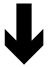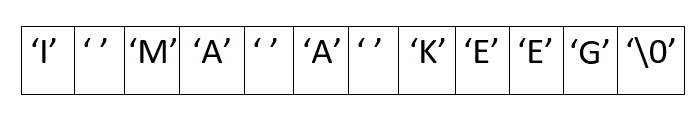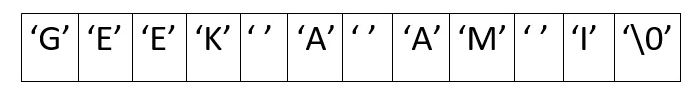The following C++ code can handle multiple contiguous spaces.

## C++

 `#include ``#include ``#include ` `using` `namespace` `std;` `string reverse_words(string s)``{``    ``int` `left = 0, i = 0, n = s.size();``  ` `    ``while` `(s[i] == ``' '``)``        ``i++;``  ` `    ``left = i;``  ` `    ``while` `(i < n)``    ``{``        ``if` `(i + 1 == n || s[i] == ``' '``)``        ``{``            ``int` `j = i - 1;``            ``if` `(i + 1 == n)``                ``j++;``          ` `            ``while` `(left < j)``                ``swap(s[left++], s[j--]);``          ` `            ``left = i + 1;``        ``}``        ``if` `(s[left] == ``' '` `&& i > left)``            ``left = i;``      ` `        ``i++;``    ``}``    ``//reverse(s.begin(), s.end());``    ``return` `s;``}` `int` `main()``{` `    ``string str = ``"I AM A GEEK"``;` `    ``str = reverse_words(str);` `    ``cout << str;``  ` `    ``return` `0;``  ``// This code is contributed``  ``// by Gatea David``}`

## Java

 `/*package whatever //do not write package name here */``import` `java.io.*;` `class` `GFG {``  ``static` `String reverse_words(String s)``  ``{``    ``int` `left = ``0``, i = ``0``, n = s.length();``    ``while` `(s.charAt(i) == ``' '``){``      ``i++;``    ``}` `    ``left = i;` `    ``while` `(i < n)``    ``{``      ``if` `(i + ``1` `== n || s.charAt(i) == ``' '``)``      ``{``        ``int` `j = i - ``1``;``        ``if` `(i + ``1` `== n)``          ``j++;` `        ``while` `(left left)``        ``left = i;` `      ``i++;``    ``}``    ``// reversing the string``    ``char` `ch[] = s.toCharArray();``    ``int` `len = s.length();``    ``for` `(i=``0``; i < (len/``2``); i++)``    ``{``      ``char` `temp = ch[i];``      ``ch[i] = ch[len - i - ``1``];``      ``ch[len - i- ``1``] = temp;``    ``}``    ``s = String.valueOf(ch);` `    ``return` `s;``  ``}` `  ``public` `static` `void` `main(String args[])``  ``{``    ``String str = ``"I AM A GEEK"``;``    ``str = reverse_words(str);``    ``System.out.println(str);``  ``}``}` `// This code is contributed by shinjanpatra.`

## Python3

 `# Python code for the same approach``def` `reverse_words(s):` `    ``left, i, n ``=` `0``, ``0``, ``len``(s)` `    ``while` `(s[i] ``=``=` `' '``):``        ``i ``+``=` `1` `    ``left ``=` `i` `    ``while` `(i < n):` `        ``if` `(i ``+` `1` `=``=` `n ``or` `s[i] ``=``=` `' '``):``        ` `            ``j ``=` `i ``-` `1``            ``if` `(i ``+` `1` `=``=` `n):``                ``j ``+``=` `1``        ` `            ``while` `(left < j):``                ``s ``=` `s[``0``:left] ``+` `s[j] ``+` `s[left``+``1``:j] ``+` `s[left] ``+` `s[j``+``1``:]``            ` `                ``left ``+``=` `1``                ``j ``-``=` `1``        ` `            ``left ``=` `i ``+` `1``        ` `        ``if` `(i > left ``and` `s[left] ``=``=` `' '``):``            ``left ``=` `i` `        ``i ``+``=` `1` `    ``s ``=` `s[::``-``1``]` `    ``return` `s` `# driver code``Str` `=` `"I AM A GEEK"``Str` `=` `reverse_words(``Str``)``print``(``Str``)` `# This code is contributed by shinjanpatra`

## C#

 `// C# program to print reverse``// of words in a string.``using` `System;` `class` `GFG {``    ``static` `String reverse_words(String s)``    ``{``        ``var` `left = 0;``        ``var` `i = 0;``        ``var` `n = s.Length;``        ``while` `(s[i] == ``' '``) {``            ``i++;``        ``}``        ``left = i;``        ``while` `(i < n) {``            ``if` `(i + 1 == n || s[i] == ``' '``) {``                ``var` `j = i - 1;``                ``if` `(i + 1 == n) {``                    ``j++;``                ``}``                ``while` `(left < n && j < n && left < j) {``                    ``char``[] ch = s.ToCharArray();``                    ``var` `temp = ch[left];``                    ``ch[left] = ch[j];``                    ``ch[j] = temp;``                    ``s = ``new` `string``(ch);``                    ``left++;``                    ``j--;``                ``}``                ``left = i + 1;``            ``}``            ``if` `(left < n && s[left] == ``' '` `&& i > left) {``                ``left = i;``            ``}``            ``i++;``        ``}``        ``// reversing the string``        ``int` `len = s.Length;``        ``char``[] chh = s.ToCharArray();``        ``for` `(i = 0; i < (len / 2); i++) {``            ``char` `temp = chh[i];``            ``chh[i] = chh[len - i - 1];``            ``chh[len - i - 1] = temp;``        ``}``        ``s = ``new` `string``(chh);``        ``return` `s;``    ``}``    ``public` `static` `void` `Main(String[] args)``    ``{``        ``string` `str = ``"I AM A GEEK"``;``        ``str = reverse_words(str);``        ``Console.WriteLine(str);``    ``}``}` `// This code is contributed by Aarti_Rathi`

## Javascript

 ``

Output:

`GEEK A AM I`

Time Complexity: O(len(str))

Auxiliary Space: O(1)

This article is contributed by MAZHAR IMAM KHAN. If you like GeeksforGeeks and would like to contribute, you can also write an article using write.geeksforgeeks.org or mail your article to review-team@geeksforgeeks.org. See your article appearing on the GeeksforGeeks main page and help other Geeks.